# User:IssaRice/Linear algebra/Change of basis example in two dimensions

This example comes from this video. To make it easier to go back and forth between this page and the video, the notation on this page tries to follow that of the video (where the discussion overlaps), but we distinguish between matrices and vectors.

We are working in$\mathbf R^2$, the plane. To be slightly pedantic, we will distinguish between matrices and vectors:$\begin{bmatrix}1 \\ 0\end{bmatrix} \in \mathbf R^{2,1}$ and$(1,0) \in \mathbf R^2$. If$v$ is a vector and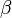$\beta$ is a basis, we write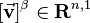$[\vec{\mathbf v}]^\beta \in \mathbf R^{n,1}$ for the "column vector" (n-by-1 matrix) and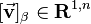$[\vec{\mathbf v}]_\beta \in \mathbf R^{1,n}$ for the "row vector" (1-by-n matrix). It's usually not necessary to be this pedantic, but here the whole point of the discussion is to understand how coordinate systems and translation between coordinate systems works, so it's worthwhile to be pedantic.

Jennifer's basis vectors: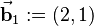$\vec{\mathbf b}_1 := (2,1)$ and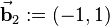$\vec{\mathbf b}_2 := (-1, 1)$.

To Jennifer,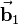$\vec{\mathbf b}_1$ looks like$(1, 0)$ and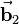$\vec{\mathbf b}_2$ looks like$(0, 1)$.

If Jennifer says "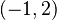$(-1, 2)$", to us (in the standard basis) this is the vector$-1\vec{\mathbf b}_1 + 2\vec{\mathbf b}_2 = -1 (2, 1) + 2(-1, 1) = (-4, 1)$.

We can also write the above calculation as$\begin{bmatrix}\uparrow & \uparrow \\ \vec{\mathbf b}_1 & \vec{\mathbf b}_2 \\ \downarrow & \downarrow\end{bmatrix}\begin{bmatrix}-1 \\ 2\end{bmatrix} = \begin{bmatrix}2 & -1 \\ 1 & 1\end{bmatrix} \begin{bmatrix}-1 \\ 2\end{bmatrix} = \begin{bmatrix}-4 \\ 1\end{bmatrix}$.

Notice that$\begin{bmatrix}\uparrow & \uparrow \\ \vec{\mathbf b}_1 & \vec{\mathbf b}_2 \\ \downarrow & \downarrow\end{bmatrix} \begin{bmatrix}\uparrow \\ \vec{\mathbf e}_1 \\ \downarrow\end{bmatrix} = \begin{bmatrix}\uparrow \\ \vec{\mathbf b}_1 \\ \downarrow\end{bmatrix}$ and$\begin{bmatrix}\uparrow & \uparrow \\ \vec{\mathbf b}_1 & \vec{\mathbf b}_2 \\ \downarrow & \downarrow\end{bmatrix} \begin{bmatrix}\uparrow \\ \vec{\mathbf e}_2 \\ \downarrow\end{bmatrix} = \begin{bmatrix}\uparrow \\ \vec{\mathbf b}_2 \\ \downarrow\end{bmatrix}$, i.e., this matrix transforms our (standard) basis vectors into Jennifer's basis vectors.

How can we write this using change of basis notation? When Jennifer says "$(-1, 2)$", this is the vector$\vec{\mathbf v} \in \mathbf R^2$ such that, when written in Jennifer's coordinate system, it has coordinates$(-1,2)$. In other words, it is the vector$v$ such that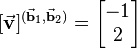$[\vec{\mathbf v}]^{(\vec{\mathbf b}_1, \vec{\mathbf b}_2)} = \begin{bmatrix}-1 \\ 2\end{bmatrix}$. To find out what this vector means in our coordinate system, we must compute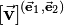$[\vec{\mathbf v}]^{(\vec{\mathbf e}_1, \vec{\mathbf e}_2)}$.

We can write$[I]_{(\vec{\mathbf b}_1, \vec{\mathbf b}_2)}^{(\vec{\mathbf e}_1, \vec{\mathbf e}_2)} [\vec{\mathbf v}]^{(\vec{\mathbf b}_1, \vec{\mathbf b}_2)} = [\vec{\mathbf v}]^{(\vec{\mathbf e}_1, \vec{\mathbf e}_2)}$. What is the meaning of the matrix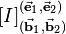$[I]_{(\vec{\mathbf b}_1, \vec{\mathbf b}_2)}^{(\vec{\mathbf e}_1, \vec{\mathbf e}_2)}$? The notation means that the columns of the matrix are Jennifer's basis vectors written using our coordinate system. It takes coordinates written in Jennifer's system and translates it into our coordinates. In other words, it translates from Jennifer's language to our language. But geometrically, it transforms our grid into Jennifer's grid. Aren't these two opposites? This is a point made in the video. The idea is to think of the matrix as transforming our misconception of what Jennifer is saying into what she is actually saying. When Jennifer says "$(1,0)$" she actually means$\vec{\mathbf b}_1$, which is the mapping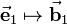$\vec{\mathbf e}_1 \mapsto \vec{\mathbf b}_1$, which, geometrically, is transforming our basis vector$\vec{\mathbf e}_1$ into Jennifer's basis vector$\vec{\mathbf b}_1$.

Now consider the linear transformation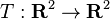$T : \mathbf R^2 \to \mathbf R^2$ defined by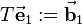$T\vec{\mathbf e}_1 := \vec{\mathbf b}_1$ and$T\vec{\mathbf e}_2 := \vec{\mathbf b}_2$. Since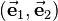$(\vec{\mathbf e}_1, \vec{\mathbf e}_2)$ is a basis of$\mathbf R^2$, there is exactly one such linear transformation, i.e., our specification is well-defined. We can check that$T$ is the map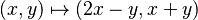$(x,y)\mapsto (2x-y, x+y)$. What is the matrix of$T$? We can look at where it takes the standard basis vectors to see that the first column is$\vec{\mathbf b}_1$ and the second column is$\vec{\mathbf b}_1$, i.e., we have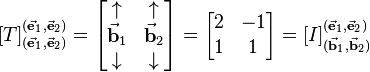$[T]_{(\vec{\mathbf e}_1, \vec{\mathbf e}_2)}^{(\vec{\mathbf e}_1, \vec{\mathbf e}_2)} = \begin{bmatrix}\uparrow & \uparrow \\ \vec{\mathbf b}_1 & \vec{\mathbf b}_2 \\ \downarrow & \downarrow\end{bmatrix} = \begin{bmatrix}2 & -1 \\ 1 & 1\end{bmatrix} = [I]_{(\vec{\mathbf b}_1, \vec{\mathbf b}_2)}^{(\vec{\mathbf e}_1, \vec{\mathbf e}_2)}$.

We should also verify that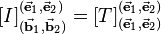$[I]_{(\vec{\mathbf b}_1, \vec{\mathbf b}_2)}^{(\vec{\mathbf e}_1, \vec{\mathbf e}_2)} = [T]_{(\vec{\mathbf e}_1, \vec{\mathbf e}_2)}^{(\vec{\mathbf e}_1, \vec{\mathbf e}_2)}$. On the one hand, the$k$th column of$[I]_{(\vec{\mathbf b}_1, \vec{\mathbf b}_2)}^{(\vec{\mathbf e}_1, \vec{\mathbf e}_2)}$ is$[I\vec{\mathbf b}_k]^{(\vec{\mathbf e}_1, \vec{\mathbf e}_2)} = [\vec{\mathbf b}_k]^{(\vec{\mathbf e}_1, \vec{\mathbf e}_2)}$. On the other hand, the$k$th column of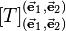$[T]_{(\vec{\mathbf e}_1, \vec{\mathbf e}_2)}^{(\vec{\mathbf e}_1, \vec{\mathbf e}_2)}$ is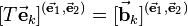$[T\vec{\mathbf e}_k]^{(\vec{\mathbf e}_1, \vec{\mathbf e}_2)} = [\vec{\mathbf b}_k]^{(\vec{\mathbf e}_1, \vec{\mathbf e}_2)}$ (the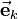$\vec{\mathbf e}_k$ comes from the basis in the subscript). So the two matrices are indeed equal.

To summarize, we can write the same equation in multiple ways:

Equation Description$-1\vec{\mathbf b}_1 + 2\vec{\mathbf b}_2 = -1 (2, 1) + 2(-1, 1) = (-4, 1)$ Linear combination of Jennifer's basis vectors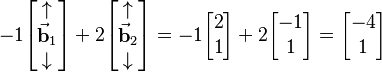$-1\begin{bmatrix}\uparrow \\ \vec{\mathbf b}_1 \\ \downarrow\end{bmatrix} + 2\begin{bmatrix}\uparrow \\ \vec{\mathbf b}_2 \\ \downarrow\end{bmatrix} = -1 \begin{bmatrix}2 \\ 1\end{bmatrix} + 2\begin{bmatrix}-1 \\ 1\end{bmatrix} = \begin{bmatrix}-4 \\ 1\end{bmatrix}$ Linear combination of Jennifer's basis vectors, written using column vectors$\begin{bmatrix}\uparrow & \uparrow \\ \vec{\mathbf b}_1 & \vec{\mathbf b}_2 \\ \downarrow & \downarrow\end{bmatrix}\begin{bmatrix}-1 \\ 2\end{bmatrix} = \begin{bmatrix}2 & -1 \\ 1 & 1\end{bmatrix} \begin{bmatrix}-1 \\ 2\end{bmatrix} = \begin{bmatrix}-4 \\ 1\end{bmatrix}$ Matrix multiplication$[I]_{(\vec{\mathbf b}_1, \vec{\mathbf b}_2)}^{(\vec{\mathbf e}_1, \vec{\mathbf e}_2)} [\vec{\mathbf v}]^{(\vec{\mathbf b}_1, \vec{\mathbf b}_2)} = [\vec{\mathbf v}]^{(\vec{\mathbf e}_1, \vec{\mathbf e}_2)}$ Change of coordinate equation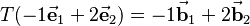$T(-1\vec{\mathbf e}_1 + 2\vec{\mathbf e}_2) = -1\vec{\mathbf b}_1 + 2\vec{\mathbf b}_2$ Application of a linear transformation to a vector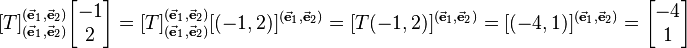$[T]_{(\vec{\mathbf e}_1, \vec{\mathbf e}_2)}^{(\vec{\mathbf e}_1, \vec{\mathbf e}_2)} \begin{bmatrix}-1 \\ 2\end{bmatrix} = [T]_{(\vec{\mathbf e}_1, \vec{\mathbf e}_2)}^{(\vec{\mathbf e}_1, \vec{\mathbf e}_2)} [(-1,2)]^{(\vec{\mathbf e}_1, \vec{\mathbf e}_2)} = [T(-1,2)]^{(\vec{\mathbf e}_1, \vec{\mathbf e}_2)} = [(-4,1)]^{(\vec{\mathbf e}_1, \vec{\mathbf e}_2)} = \begin{bmatrix}-4 \\ 1\end{bmatrix}$ Matrix of linear transformation in standard coordinates